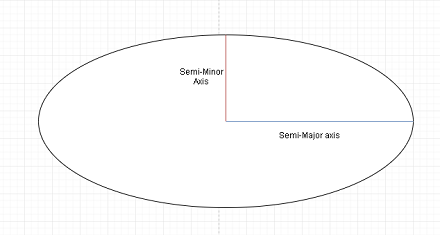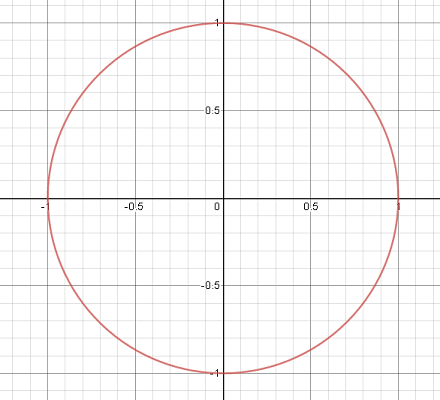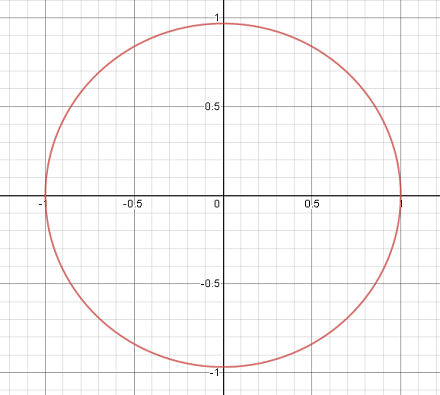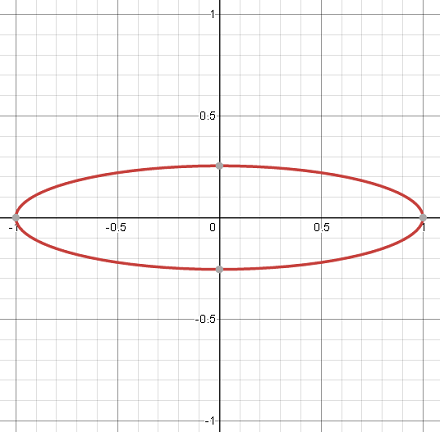# Pluto's orbit is more eccentric than any other of the major planets. What does that mean?

## Question:

Pluto's orbit is more eccentric than any other of the major planets. What does that mean?

## Eccentricity

Eccentricity is a parameter of an ellipse which describes how "squished" or "flattened" the ellipse is when compared to a circle.

An ellipse has two different "radii", a longer one called the semi-major axis and a shorter one called the semi-minor axis, pictured below.The eccentricity is usually denoted e and takes a number between 0 and 1 for an ellipse. It can be calculated as:

{eq}\displaystyle e = \frac{\sqrt{a^2-b^2}}{a} {/eq}

Here a is the length of the semi-major axis and b is the length of the semi-minor axis.

In this sense the eccentricity is a measure of how much bigger the semi-major axis is then the semi-minor axis. In the case of a circle e=0 since the semi-major and semi-minor axis have the same length.

Pluto's orbit being called more eccentric than the other major planets simply refers to how elliptical Pluto's orbit is.

Since eccentricity is a measure of how "flattened" an ellipse is, Pluto being more eccentric simply means that it has a bigger difference in the length of its semi-major and semi-minor axes than the other planet's orbits.

For example, the Earth's orbit is very close to a circle, with an eccentricity of about 0.017 (a circle has eccentricity zero). For a visual reference this looks like:This graph is quite accurate to scale, and is basically indistinguishable from a circle.

For Pluto's orbit however, with eccentricity of about 0.248 this looks like:This still looks quite a lot like a circle but it is much more obvious that it is not. Notice how the vertical part of the circle comes a little short of 1 while the horizontal portion goes to exactly 1, showing that it is indeed elliptical.

As a more extreme example, Halley's comet which is comet which orbits the Sun has an eccentricity of 0.967 would have an orbit which looks like:So when Pluto's orbit is referred to as being eccentric it is referring to this fact. That the shape of Pluto's orbit is more like an ellipse than the other major planet's orbits are, even though they are all still quite circular.